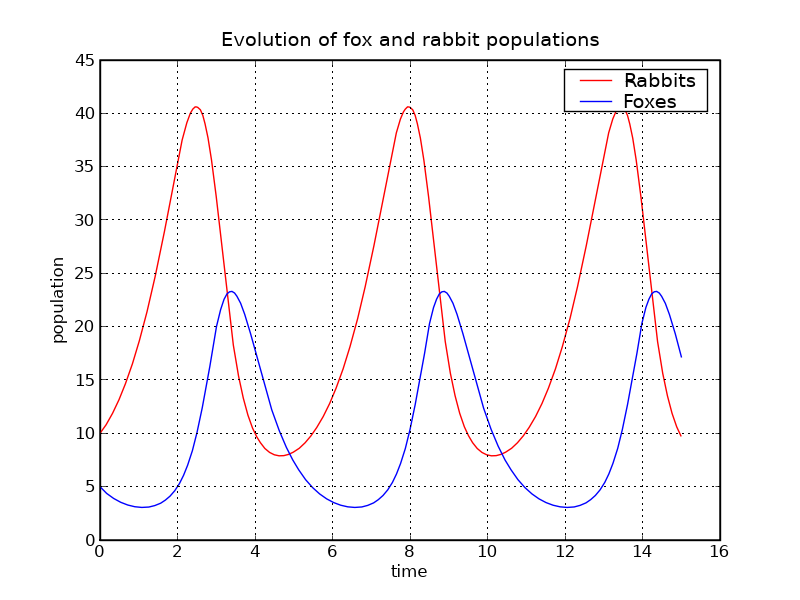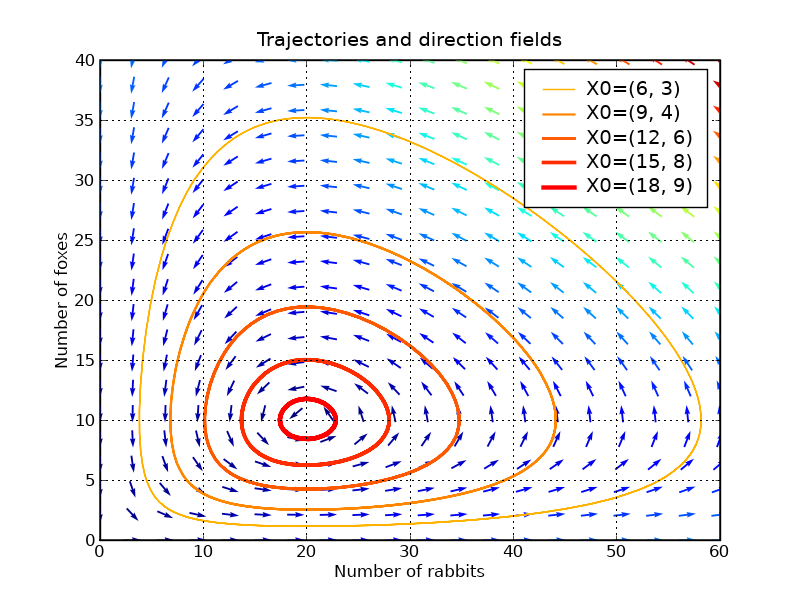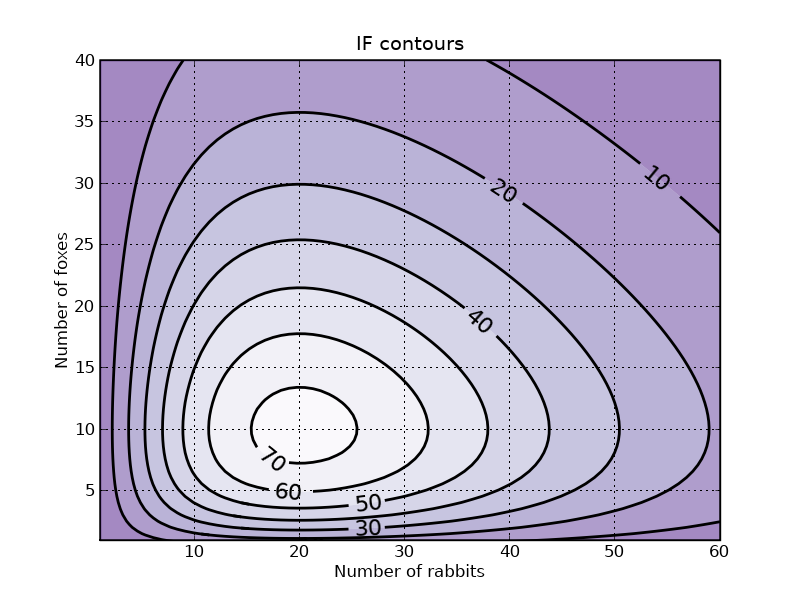This is an archival dump of old wiki content --- see scipy.org for current material.

This example describes how to integrate ODEs with the scipy.integrate module, and how to use the matplotlib module to plot trajectories, direction fields and other information.

You can get the source code for this tutorial here: tutorial_lokta-voltera_v4.py .

## Presentation of the Lotka-Volterra Model

We will have a look at the Lotka-Volterra model, also known as the predator-prey equations, which is a pair of first order, non-linear, differential equations frequently used to describe the dynamics of biological systems in which two species interact, one a predator and the other its prey. The model was proposed independently by Alfred J. Lotka in 1925 and Vito Volterra in 1926, and can be described by

```du/dt =  a*u -   b*u*v
dv/dt = -c*v + d*b*u*v```

with the following notations:

• u: number of preys (for example, rabbits)
• v: number of predators (for example, foxes)
• a, b, c, d are constant parameters defining the behavior of the population:
• a is the natural growing rate of rabbits, when there's no fox
• b is the natural dying rate of rabbits, due to predation
• c is the natural dying rate of fox, when there's no rabbit
• d is the factor describing how many caught rabbits let create a new fox

We will use X=[u, v] to describe the state of both populations.

Definition of the equations:

```   1 from numpy import *
2 import pylab as p
3 # Definition of parameters
4 a = 1.
5 b = 0.1
6 c = 1.5
7 d = 0.75
8 def dX_dt(X, t=0):
9     """ Return the growth rate of fox and rabbit populations. """
10     return array([ a*X -   b*X*X ,
11                   -c*X + d*b*X*X ])
```

### Population equilibrium

Before using SciPy to integrate this system, we will have a closer look at position equilibrium. Equilibrium occurs when the growth rate is equal to 0. This gives two fixed points:

```   1 X_f0 = array([     0. ,  0.])
2 X_f1 = array([ c/(d*b), a/b])
3 all(dX_dt(X_f0) == zeros(2) ) and all(dX_dt(X_f1) == zeros(2)) # => True
```

### Stability of the fixed points

Near these two points, the system can be linearized: dX_dt = A_f*X where A is the Jacobian matrix evaluated at the corresponding point. We have to define the Jacobian matrix:

```   1 def d2X_dt2(X, t=0):
2     """ Return the Jacobian matrix evaluated in X. """
3     return array([[a -b*X,   -b*X     ],
4                   [b*d*X ,   -c +b*d*X] ])
```

So near X_f0, which represents the extinction of both species, we have:

```   1 A_f0 = d2X_dt2(X_f0)                    # >>> array([[ 1. , -0. ],
2                                         #            [ 0. , -1.5]])
```

Near X_f0, the number of rabbits increase and the population of foxes decrease. The origin is therefore a saddle point.

Near X_f1, we have:

```   1 A_f1 = d2X_dt2(X_f1)                    # >>> array([[ 0.  , -2.  ],
2                                         #            [ 0.75,  0.  ]])
3 # whose eigenvalues are +/- sqrt(c*a).j:
4 lambda1, lambda2 = linalg.eigvals(A_f1) # >>> (1.22474j, -1.22474j)
5 # They are imaginary numbers. The fox and rabbit populations are periodic as follows from further
6 # analysis. Their period is given by:
7 T_f1 = 2*pi/abs(lambda1)                # >>> 5.130199
```

## Integrating the ODE using scipy.integrate

Now we will use the scipy.integrate module to integrate the ODEs. This module offers a method named odeint, which is very easy to use to integrate ODEs:

```   1 from scipy import integrate
2 t = linspace(0, 15,  1000)              # time
3 X0 = array([10, 5])                     # initials conditions: 10 rabbits and 5 foxes
4 X, infodict = integrate.odeint(dX_dt, X0, t, full_output=True)
5 infodict['message']                     # >>> 'Integration successful.'
```

infodict is optional, and you can omit the full_output argument if you don't want it. Type "info(odeint)" if you want more information about odeint inputs and outputs.

We can now use Matplotlib to plot the evolution of both populations:

```   1 rabbits, foxes = X.T
2 f1 = p.figure()
3 p.plot(t, rabbits, 'r-', label='Rabbits')
4 p.plot(t, foxes  , 'b-', label='Foxes')
5 p.grid()
6 p.legend(loc='best')
7 p.xlabel('time')
8 p.ylabel('population')
9 p.title('Evolution of fox and rabbit populations')
10 f1.savefig('rabbits_and_foxes_1.png')
```The populations are indeed periodic, and their period is close to the value T_f1 that we computed.

## Plotting direction fields and trajectories in the phase plane

We will plot some trajectories in a phase plane for different starting points between X_f0 and X_f1.

We will use Matplotlib's colormap to define colors for the trajectories. These colormaps are very useful to make nice plots. Have a look at ShowColormaps if you want more information.

```   1 values  = linspace(0.3, 0.9, 5)                          # position of X0 between X_f0 and X_f1
2 vcolors = p.cm.autumn_r(linspace(0.3, 1., len(values)))  # colors for each trajectory
3
4 f2 = p.figure()
5
6 #-------------------------------------------------------
7 # plot trajectories
8 for v, col in zip(values, vcolors):
9     X0 = v * X_f1                               # starting point
10     X = integrate.odeint( dX_dt, X0, t)         # we don't need infodict here
11     p.plot( X[:,0], X[:,1], lw=3.5*v, color=col, label='X0=(%.f, %.f)' % ( X0, X0) )
12
13 #-------------------------------------------------------
14 # define a grid and compute direction at each point
15 ymax = p.ylim(ymin=0)                        # get axis limits
16 xmax = p.xlim(xmin=0)
17 nb_points   = 20
18
19 x = linspace(0, xmax, nb_points)
20 y = linspace(0, ymax, nb_points)
21
22 X1 , Y1  = meshgrid(x, y)                       # create a grid
23 DX1, DY1 = dX_dt([X1, Y1])                      # compute growth rate on the gridt
24 M = (hypot(DX1, DY1))                           # Norm of the growth rate
25 M[ M == 0] = 1.                                 # Avoid zero division errors
26 DX1 /= M                                        # Normalize each arrows
27 DY1 /= M
28
29 #-------------------------------------------------------
30 # Drow direction fields, using matplotlib 's quiver function
31 # I choose to plot normalized arrows and to use colors to give information on
32 # the growth speed
33 p.title('Trajectories and direction fields')
34 Q = p.quiver(X1, Y1, DX1, DY1, M, pivot='mid', cmap=p.cm.jet)
35 p.xlabel('Number of rabbits')
36 p.ylabel('Number of foxes')
37 p.legend()
38 p.grid()
39 p.xlim(0, xmax)
40 p.ylim(0, ymax)
41 f2.savefig('rabbits_and_foxes_2.png')
```This graph shows us that changing either the fox or the rabbit population can have an unintuitive effect. If, in order to decrease the number of rabbits, we introduce foxes, this can lead to an increase of rabbits in the long run, depending on the time of intervention.

## Plotting contours

We can verify that the function IF defined below remains constant along a trajectory:

```   1 def IF(X):
2     u, v = X
3     return u**(c/a) * v * exp( -(b/a)*(d*u+v) )
4 # We will verify that IF remains constant for different trajectories
5 for v in values:
6     X0 = v * X_f1                               # starting point
7     X = integrate.odeint( dX_dt, X0, t)
8     I = IF(X.T)                                 # compute IF along the trajectory
9     I_mean = I.mean()
10     delta = 100 * (I.max()-I.min())/I_mean
11     print 'X0=(%2.f,%2.f) => I ~ %.1f |delta = %.3G %%' % (X0, X0, I_mean, delta)
12 # >>> X0=( 6, 3) => I ~ 20.8 |delta = 6.19E-05 %
13 #     X0=( 9, 4) => I ~ 39.4 |delta = 2.67E-05 %
14 #     X0=(12, 6) => I ~ 55.7 |delta = 1.82E-05 %
15 #     X0=(15, 8) => I ~ 66.8 |delta = 1.12E-05 %
16 #     X0=(18, 9) => I ~ 72.4 |delta = 4.68E-06 %
```

Plotting iso-contours of IF can be a good representation of trajectories, without having to integrate the ODE

```   1 #-------------------------------------------------------
2 # plot iso contours
3 nb_points = 80                              # grid size
4 x = linspace(0, xmax, nb_points)
5 y = linspace(0, ymax, nb_points)
6 X2 , Y2  = meshgrid(x, y)                   # create the grid
7 Z2 = IF([X2, Y2])                           # compute IF on each point
8 f3 = p.figure()
9 CS = p.contourf(X2, Y2, Z2, cmap=p.cm.Purples_r, alpha=0.5)
10 CS2 = p.contour(X2, Y2, Z2, colors='black', linewidths=2. )
11 p.clabel(CS2, inline=1, fontsize=16, fmt='%.f')
12 p.grid()
13 p.xlabel('Number of rabbits')
14 p.ylabel('Number of foxes')
15 p.ylim(1, ymax)
16 p.xlim(1, xmax)
17 p.title('IF contours')
18 f3.savefig('rabbits_and_foxes_3.png')
19 p.show()
```SciPy: Cookbook/LoktaVolterraTutorial (last edited 2015-10-24 17:48:23 by anonymous)